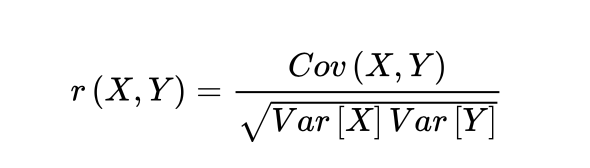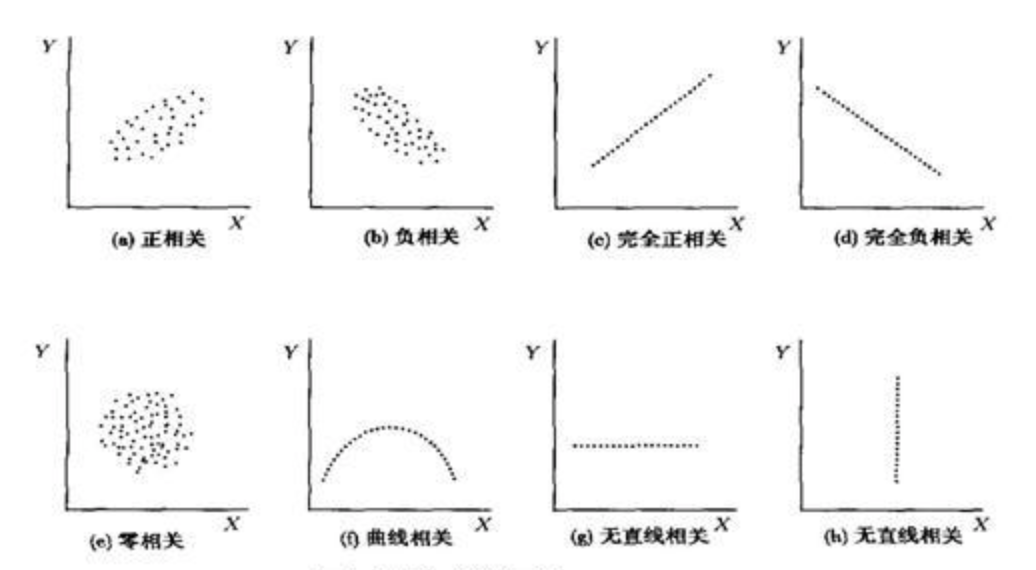Robinx

V1

2021/04/09阅读：138主题：草原绿

# 工业蒸汽预测baseline-数据探索篇

## 赛题数据

``# 数据探索import numpy as npimport pandas as pd import matplotlib.pyplot as plt import seaborn as sns from scipy import statsimport warningswarnings.filterwarnings("ignore")%matplotlib inline# 载入训练集与数据集train_data_file = "./zhengqi_train.txt"test_data_file = "./zhengqi_test.txt"# 读取数据train_data = pd.read_csv(train_data_file,sep='\t',encoding='utf-8')test_data = pd.read_csv(test_data_file,sep='\t',encoding='utf-8')# 查看集合的所有特征数据大撒东方大厦train_data.head(10)test_data.head(10)``

## 评估指标

``from sklearn.metrics import mean_squared_errormean_squared_error(y_test,y_predict)``

## 数据分析

• 绘制散点图，观察变量之间是线性还是非线性
• 计算相关性，相关性公式如下相关系数的取值范围为[-1,1],为-1时，表示强负线性相关，为1时表示正线性相关，为0，表示不相关使用numpy库中的corrcoef函数可以对两种变量进行相关性检测

• 直接删除数据，这样很简单，但是会使样本减少，拟合能力降低
• 均值，模型，中值填充
• 预测模型填充

``fig = plt.figure(figsize=(4,6)) # 绘制图形sns.boxplot(train_data['V0'],orient="v",width=0.5)``

``# 绘制每一列的箱线图column = train_data.columns.tolist()[:39]fig = plt.figure(figsize=(80,60),dpi=75)for i in range(38):    plt.subplot(7,8,i+1)    sns.boxplot(train_data[column[i]],orient="v",width=0.5)    plt.ylabel(column[i],fontsize=36)plt.show()``

``# 直方图的绘制plt.figure(figsize=(10,5))ax = plt.subplot(1,2,1)sns.distplot(train_data['V0'],fit=stats.norm)ax = plt.subplot(1,2,2)res = stats.probplot(train_data['V0'],plot=plt)``

``# 绘制所有正太分布图train_cols = 6train_rows = len(train_data.columns)plt.figure(figsize=(4 * train_cols,4 * train_rows))i = 0for col in train_data.columns:    i = i + 1    ax = plt.subplot(train_rows,train_cols,i)     sns.distplot(train_data[col],fit=stats.norm)    i = i + 1    ax = plt.subplot(train_rows,train_cols,i)     res = stats.probplot(train_data[col],plot=plt)plt.tight_layout()plt.show()``

``plt.figure(figsize=(8,4),dpi=150)ax = sns.kdeplot(train_data['V0'],color='Red',shade=True)ax = sns.kdeplot(test_data['V0'],color="blue",shade=True)ax.set_xlabel('V0')ax.set_ylabel("Frequency")ax = ax.legend(["train","test"])``

``# 查看所有特征与target变量之间的线性关系fcols = 6frows = len(test_data.columns)plt.figure(figsize=(5 * fcols,4 * frows))i = 0for col in test_data.columns:    i = i + 1    ax = plt.subplot(frows,fcols,i)     sns.regplot(x=col,y='target',data=train_data,ax=ax,scatter_kws={'marker':'.','s':3,'alpha':0.3},line_kws={'color':'k'});    plt.xlabel(col)    plt.ylabel('target')    i = i + 1    ax = plt.subplot(frows,fcols,i)    sns.distplot(train_data[col].dropna())    plt.xlabel(col)``

``# 删除无关的特征变量pd.set_option('display.max_columns',10)pd.set_option('display.max_rows',10)data_train1 = train_data.drop(['V5','V9','V11','V17','V22','V28'],axis = 1)train_corr = data_train1.corr()train_corr``

``# 找出与target相关性最相关的特征 变量k = 10cols = train_corr.nlargest(k,'target')['target'].indexcm = np.corrcoef(train_data[cols].values.T)hm = plt.subplots(figsize=(10,10)) #调整画布大小hm = sns.heatmap(train_data[cols].corr(),annot=True,square=True)plt.show()``

``# 筛选出特征变量大于0.5的特征变量threshold = 0.5corrmat = train_data.corr()top_corr_features = corrmat.index[abs(corrmat['target']) > threshold]plt.figure(figsize=(10,10))g = sns.heatmap(train_data[top_corr_features].corr(),annot=True,cmap="RdYlGn")``

``# 移除相关特征threshold = 0.5# 相关系数矩阵corr_matrix = data_train1.corr().abs()drop_col=corr_matrix[corr_matrix["target"] < threshold].index data_all.drop(drop_col,axis=1,inplace=True)``

## 数据转换

``# Box-Cox变换drop_columns = ['V5','V9','V11','V22','V28']# 合并训练集和测试集的数据train_X = train_data.drop(['target'],axis=1)data_all = pd.concat([train_data,test_data],axis=0,ignore_index=True)data_all.drop(drop_columns,axis=1,inplace=True)data_all.head()``
``# 对合并后的每列数据进行归一化cols_numeric=list(data_all.columns)def scale_minmax(col):    return (col-col.min()) / (col.max()-col.min())data_all[cols_numeric] = data_all[cols_numeric].apply(scale_minmax,axis=0)data_all[cols_numeric].describe()``

``train_data_process = train_data[cols_numeric]train_data_process = train_data_process[cols_numeric].apply(scale_minmax,axis=0)test_data_process = test_data[cols_numeric]test_data_process = test_data_process[cols_numeric].apply(scale_minmax,axis=0)``

## 5.3 赛题资料V1